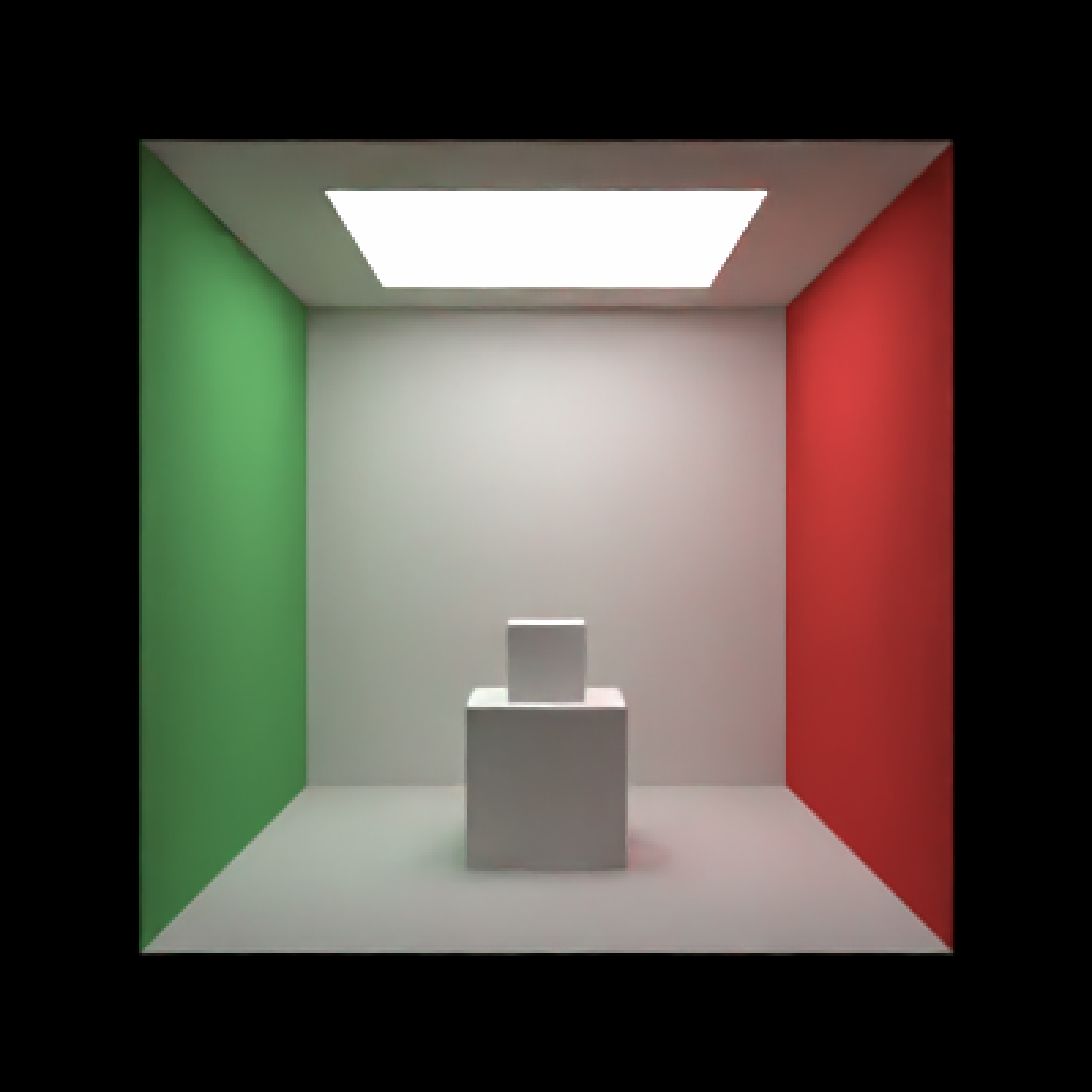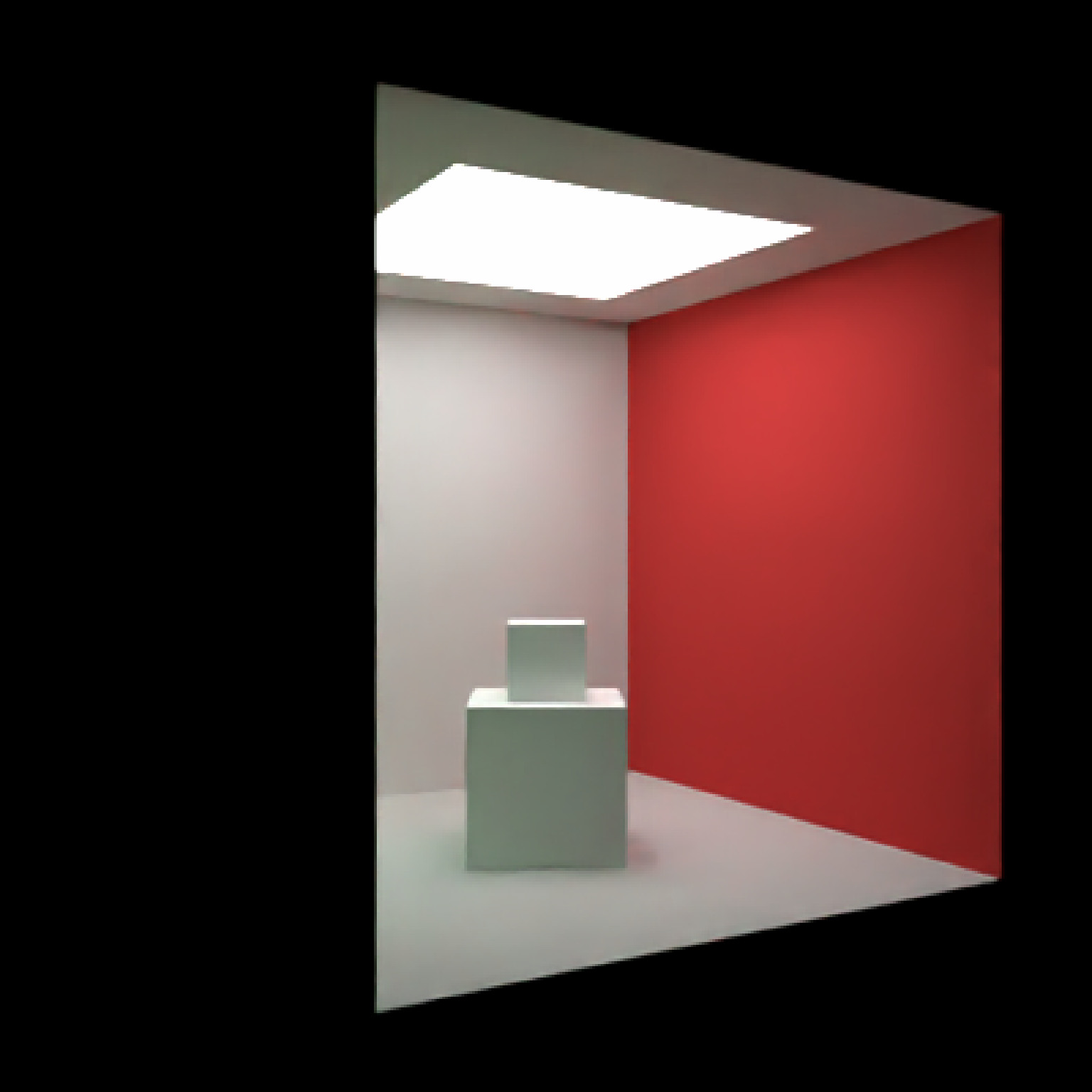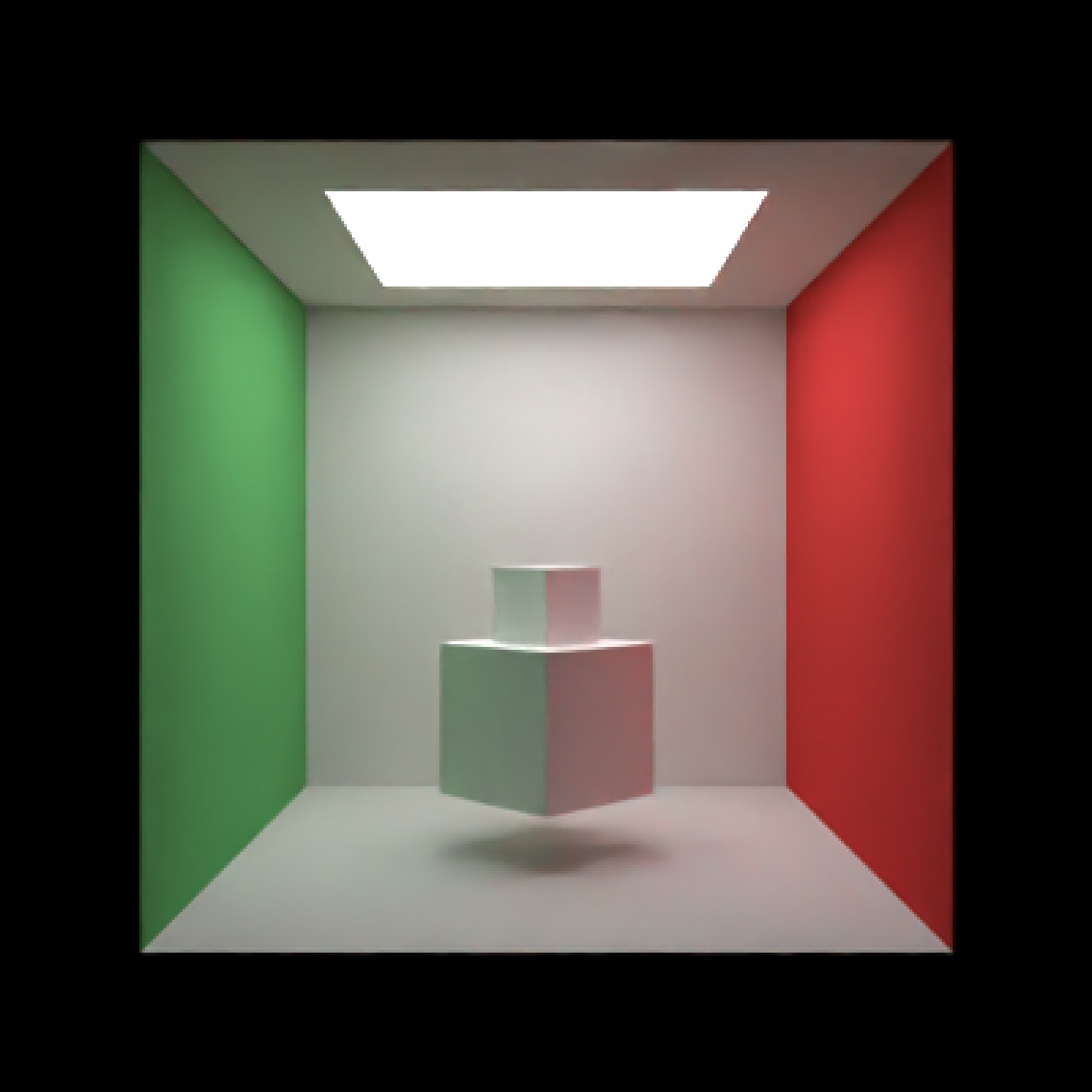Group and transform objects together. Currently only supports a single level of grouping.

group_objects(
scene,
pivot_point = c(0, 0, 0),
group_translate = c(0, 0, 0),
group_angle = c(0, 0, 0),
group_order_rotation = c(1, 2, 3),
group_scale = c(1, 1, 1)
)

## Arguments

scene Tibble of pre-existing object locations and properties to group together. Defaults to the mean location of all the objects. The point about which to pivot and move the group. Default c(0,0,0). Vector indicating where to offset the group. Default c(0,0,0). Angle of rotation around the x, y, and z axes, applied in the order specified in order_rotation. Default c(1,2,3). The order to apply the rotations, referring to "x", "y", and "z". Default c(1,1,1). Scaling factor for x, y, and z directions for all objects in group.

## Value

Tibble of grouped object locations and properties.

## Examples

#Generate the ground and add some objects
scene = generate_cornell() %>%
# \donttest{
render_scene(scene,lookfrom=c(278,278,-800),lookat = c(278,278,0), aperture=0,
samples=500, fov=50, parallel=TRUE, clamp_value=5)# }

#Group the entire room and rotate around its center, but keep the cubes in the same place.
scene2 = group_objects(generate_cornell(),
pivot_point=c(555/2,555/2,555/2),
group_angle=c(0,30,0)) %>%

# \donttest{
render_scene(scene2,lookfrom=c(278,278,-800),lookat = c(278,278,0), aperture=0,
samples=500, fov=50, parallel=TRUE, clamp_value=5)# }

#Now group the cubes instead of the Cornell box, and rotate/translate them together
twocubes = cube(x=555/2,y=555/8,z=555/2,width=555/4) %>%
add_object(cube(x=555/2, y=555/4 + 555/16, z=555/2, width=555/8))
scene3 = generate_cornell() %>%
add_object(group_objects(twocubes, group_translate = c(0,50,0),group_angle = c(0,45,0)))
# \donttest{
render_scene(scene3,lookfrom=c(278,278,-800),lookat = c(278,278,0), aperture=0,
samples=500, fov=50, parallel=TRUE, clamp_value=5)# }

#Flatten and stretch the cubes together on two axes
scene4 = generate_cornell() %>%# }